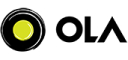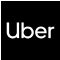New update is available. Click here to update.
Topics

# K Most Frequent Elements

Moderate0/80
Average time to solve is 10m+12 more companies

## Problem statement

You are given an Integer array ‘ARR’ and an Integer ‘K’.

Your task is to find the ‘K’ most frequent elements in ‘ARR’. Return the elements in any order.

For Example:

``````You are given ‘ARR’ = {1, 2, 2, 3, 3} and ‘K’ = 2.

The answer will {2, 3} as 2 and 3 are the elements occurring most times.
``````
Detailed explanation ( Input/output format, Notes, Images )
Sample Input 1:
``````5 2
1 2 2 3 3
``````
Sample Output 1:
``````2 3
``````
Explanation of Sample Input 1:
``````The answer will {2, 3} as 2 and 3 are the elements occurring the most number of times.
``````
Sample Input 2:
``````2 2
1 2
``````
Sample Output 2:
``````1 2
``````
Constraints:
``````1 <= 'N' <= 10^5
1 <= 'K' <= Number of unique elements in ‘ARR’
1 <= 'ARR[i]' <= 10^6

Time Limit: 1 sec
``````Console